Refer to our Texas Go Math Grade 1 Answer Key Pdf to score good marks in the exams. Test yourself by practicing the problems from Texas Go Math Grade 1 Lesson 4.5 Answer Key Add in Any Order.

Essential Question
Explanation:
Addends are nothing but the partners
WE can add them in any order.

Explore
Use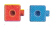to model the addition sentence. Draw to show your work.Explanation:
2 + 3 = 5
and
3 + 2 = 5
but the product is same.

For The Teacher

• Direct children to do the following. Use connecting çubes to show 2 + 3 and then 3 + 2.Explanation:
2 + 3 = 5
and
3 + 2 = 5
but the product is same.

Math Talk
Mathematical processes
How is 2 + 3 = 5 the same as 3 + 2 = 5? Explain how it is different.
Explanation:
Both the sums are same
2, 3 are partners of 5

Model and Draw
The order of the addends changes. What do you notice about the sum?Explanation: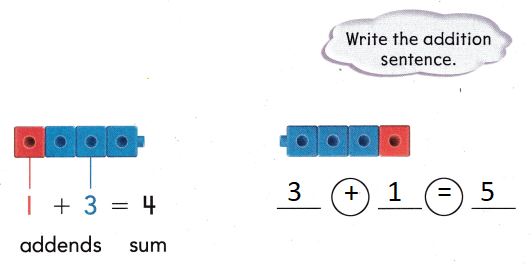The order of addends is different but the sum does not changed

Share and Show

Use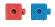to add. Color to match. Write the sum.

Change the order of the addends. Color to match. Write the addition sentence.

Question 1.Explanation:
2 + 3 = 5
and
3 + 2 = 5
but the sum is same.

Question 2.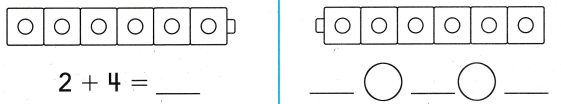Explanation:
2 + 4 = 6
4 + 2 = 6
but the sum is same.

Question 3.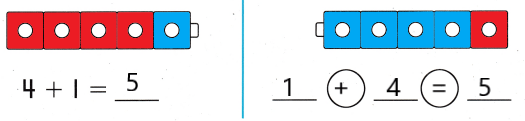Explanation:
but the sum is same.
4 + 1 = 5
1 + 4 = 5

Problem Solving

UseWrite the sum. Circle the addition sentences in each row that have the same addends in a different order.

Question 4.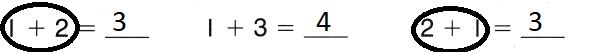Explanation:
1 + 2 = 3
2 + 1 = 3

Question 5.Explanation:
1 + 5 = 6
4 + 2 = 6
2 + 4 = 6

Question 6.
Multi-Step Choose addends to complete the addition sentence. Change the order. Write the numbers.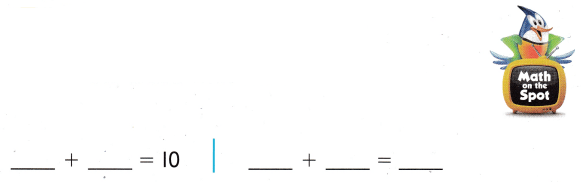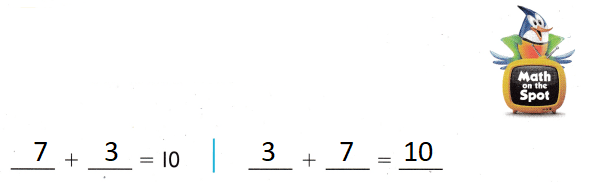Explanation:
7 + 3 = 10
3 + 7 = 10

Question 7.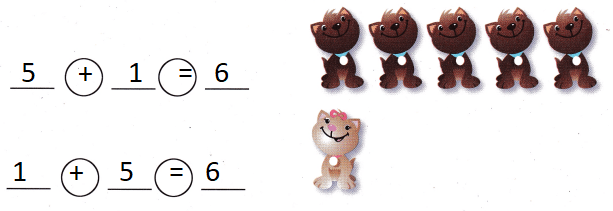Explanation:
5 + 1 = 6
1 + 5 = 6

Question 8.
The picture shows Omar’s shells. The addition sentence 5 + 3 = 8 tells about the picture. What other addition sentence tells about the picture?
(A) 3 + 3 = 6
(B) 5 + 2 = 7
(C) 3 + 5 = 8
Explanation:
5 + 3 = 8
3 + 5 = 8
but the sum is same.

Question 9.
Analyze Pam knows that 4 + 1 = 5. Which other addends have a sum of 5?
(A) 1 + 4
(B) 5 + 1
(C) 4 + 5
Explanation:
4 + 1 = 5
1 + 4 = 5
but the sum is same.

Question 10.
Gabe wrote 4 + 3 = 7. He put the addends in a different order. Which is the addition sentence he wrote?
(A) 2 + 5 = 7
(B) 3 + 4 = 7
(C) 3 + 7 = 10
Explanation:
4 + 3 = 7
3 + 4 = 7
but the sum is same.

Question 11.
Texas Test Prep Which shows the same addends in a different order?
2 + 3 = 5
(A) 4 + 1 = 5
(B) 3 + 5 = 8
(C) 3 + 2 = 5
Explanation:
2 + 3 = 5
3 + 2 = 5
but the sum is same.

Take Home Activity

• Ask your child to use small objects of the same kind to show 2 + 4 and 4 + 2, and then explain to you why the sums are the same. Repeat with other addition sentences.Explanation:
2 + 4 = 6
4 + 2 = 6
but the sum is same.

### Texas Go Math Grade 1 Lesson 4.5 Homework and Practice Answer Key

Use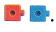Write the sums. Circle the addition sentences in each row that have the same addends in a different order.

Question 1.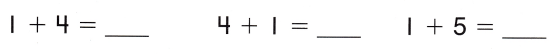Explanation:
1 + 4 = 5
4 + 1 = 5
but the sum is same.

Question 2.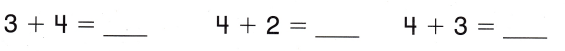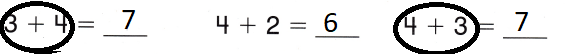Explanation:
but the sum is same.
4 + 3 = 7
3 + 4 = 7

Problem Solving

Write or draw to solve.

Question 3.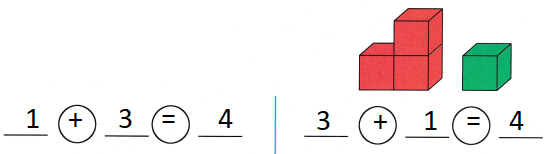Explanation:
3 + 1 = 4
1 + 3 = 4
but the sum is same.

Question 4.
Multi-Step Choose addends to complete the addition sentence. Change the order. Write the numbers.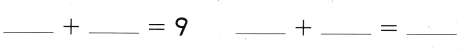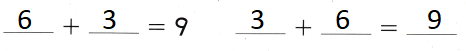Explanation:
6 + 3 = 9
3 + 6 = 9
but the sum is same.

Texas Test Prep

Lesson Check

Question 5.
The picture shows Lexi’s pets. The addition sentence 3 + 6 = 9 tells about the picture. What other addition sentence tells about the picture?(A) 6 + 4 = 10
(B) 6 + 3 = 9
(C) 3 + 5 = 8
Explanation:
3 + 6 = 9
6 + 3 = 9
but the sum is same.

Question 6.
Colin writes 2 + 1 = 3. Which other addends could he write that have a sum of 3?
(A) 3 + 1
(B) 1 + 2
(C) 2 + 3
Explanation:
1 + 2 = 3
2 + 1 = 3
but the sum is same.

Question 7.
Which shows the same addends in a different order?
4 + 2 = 6
(A) 4 + 1 = 5
(B) 2 + 6 = 8
(C) 2 + 4 = 6
Explanation:
4 + 2 = 6
2 + 4 = 6
but the sum is same.

Question 8.
Carly wrote 6 + 4=10. Then she put the addends in a different order. Which of these could be the addition sentence she wrote?
(A) 4 + 6 = 10
(B) 3 + 7 = 10
(C) 4 + 2 = 10